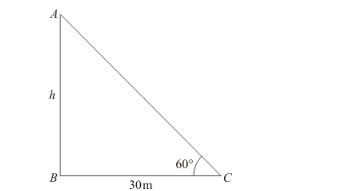# If the altitude of the sum is at 60°,

Question:

If the altitude of the sum is at 60°, then the height of the vertical tower that will cast a shadow of length 30 m is

(a) $10 \sqrt{3} \mathrm{~m}$

(b) $15 \mathrm{~m}$

(c) $\frac{30}{\sqrt{3}} m$

(d) $15 \sqrt{2} \mathrm{~m}$

Solution:

Letbe the height of vertical towerGiven that: altitude of sun isand shadow of lengthmeters.

Here, we have to find the height of tower.

So we use trigonometric ratios.

In a triangle,

$\Rightarrow \tan C=\frac{A B}{B C}$

$\Rightarrow \tan 60^{\circ}=\frac{A B}{B C}$

$\Rightarrow \sqrt{3}=\frac{h}{30}$

$\Rightarrow h=30 \sqrt{3}$
Hence the correct option is $a$.